# Mathematics - All About Geometry (Part 1)

in StemSocial2 months ago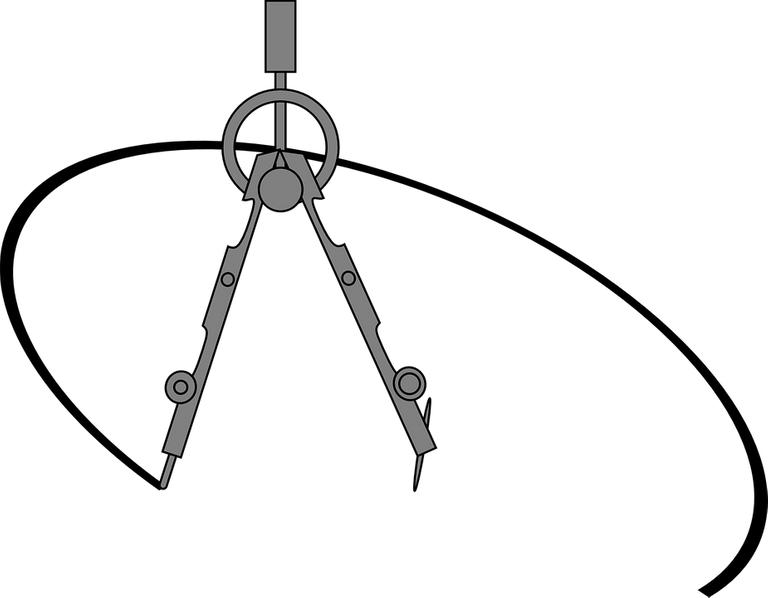[Image1]

## Introduction

Hey it's a me again @drifter1!

This article marks the beginning of a small series, where we will try to cover all the important things about Geometry. A small refresher on high-school mathematics, for anyone that might still have problems with some of the topics. In this first part we will get started with the main concepts of Geometry.

So, without further ado, let's get straight into it!

## What is Geometry?

Geometry is a branch of mathematics which deals with the shape, size and properties of individual objects, as well as the relationship amongst the points, lines, angles, surfaces and solids that make up those objects. It's one of the oldest branches of mathematics around, and its name is derived from the Ancient Greek words: γῆ (earth) and μέτρον (to measure). Thus, Geometry basically means "measuring the Earth".

Eventually, the Euclidean geometry, which only studied flat surfaces (plane geometry) and rigid three-dimensional objects (solid geometry) evolved and split into even more branches. Thus, nowadays we are talking about non-Euclidian geometrical branches such as: analytic, algebraic, computational, discrete, projective and differential geometry, or the various topology branches. Each and everyone of them deals with specific topics and problems.

## The Fundamentals: Points, Lines, Planes and Solids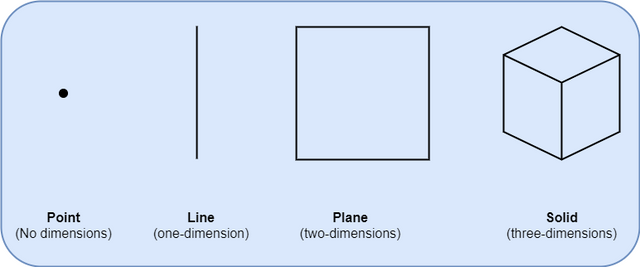In Geometry we define the following:

• Point: No dimension, only position
• Line: One-dimensional - connects two points
• Plane: Two-dimensionsal (2D) - specified by width and height
• Solid: Three-dimensional (3D) - specified by width, depth and height

## The 5 Axioms of Geometry

All mathematical branches are built on a specific mathematical ruleset.
In geomerty these rules are referred to as axioms, and there are 5 of them.

### 1. A straight line can be drawn between any two points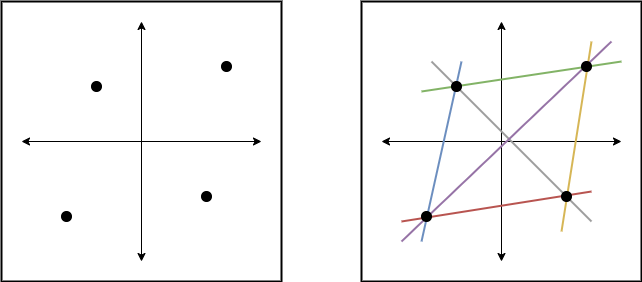Any two points on the same plane (flat space) can be connected by a straight line. In other words all "dots" can be connected.

### 2. A straight line can be extended indefinitely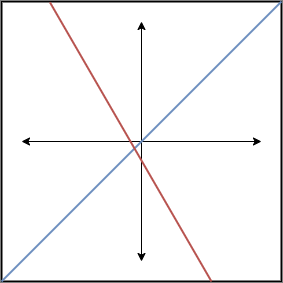A line is not limited to the paper its drawn on, it can reach to infinity!

### 3. A circle can be made using any point as its center and a line segment as its radius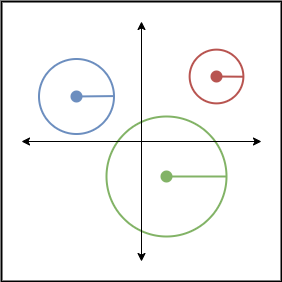So, any point can be used in order to make a circle, and the radius of that circle will always be a straight line from the center to the edge of the circle.

### 4. All right angles are congruent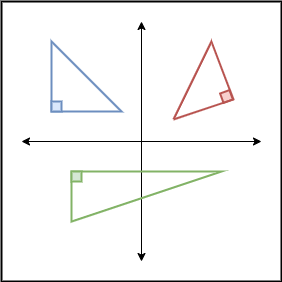Congruence means that all right angles are the same. A right angle is thus always 90° or π / 2 radians.

### 5. Parallel postulate: Given a straight line and a point which is not on the line, there is always a second straight line through the point that will be parallel to the first line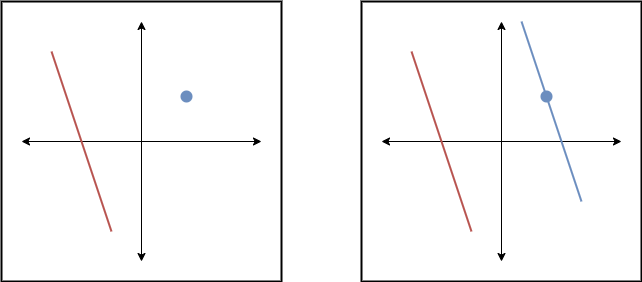To simplify this, parallel postulate basically means that we can always draw a second line which will be parallel to another line, if both are on the same plane.

## Plane Geometry and 2D Shapes

Plane geometry, is the subset of geometry which is all about shapes in a flat surface (a somewhat endless piece of paper). Flat or 2D shapes are things such as squares, circles and triangles. This basically includes any polygon and curved shape that can be drawn on a piece of paper.

### Polygons

Polygons are defined by the sides (or edges) and corners (or vertices).
There is a special class of them, the Regular Polygons, where all the sides and angles are the same.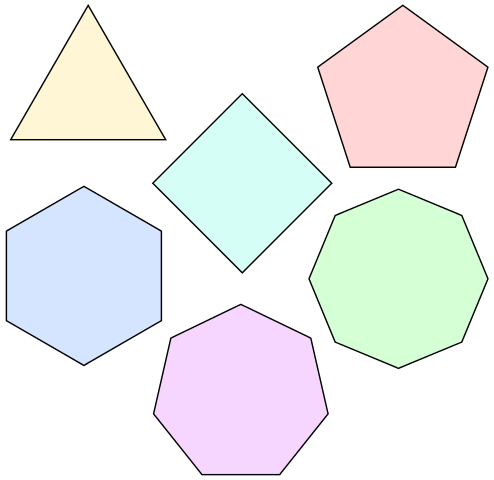[Image 2]

These are called:

• Triangle - 3 Sides
• Square - 4 Sides
• Pentagon - 5 Sides
• Hexagon - 6 sides
• Heptagon - 7 sides
• Octagon - 8 sides

etc.

Any polygon satisfies the following property: its exterior angles add up to 360°. So, for any regular polygon each exterior angle is 360° / n, where n is the number of sides. Similarly, the interior angles always add up to 180°. Thus, the relation of the angles is:

Interior Angle = 180° - Exterior Angle

which in the case of regular polygons leads to the relation:

Interior Angle = 180° - 360° / n

Common polygon shapes also include shapes such as the Quadrilateral and Rectangle, which both have 4 sides. The first specifies any 4 sized 2D shape, whilst the second has all right angles.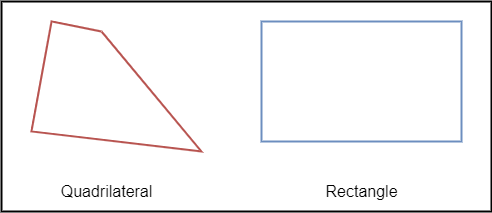### Curved Shapes

Let's not forget the curved shapes, which are shapes such as the circle and ellipse.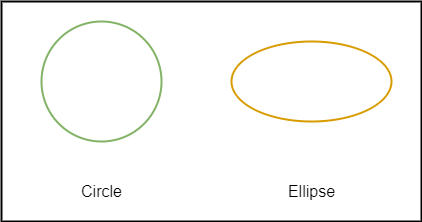### Triangles

Triangles have three sides and three angles, and the interior angles always add up to 180° or π radians.
Depending on their shape they can be given special names:

• Equilateral Triangle: Three equal sides and three equal angles (each angle is 60°).
• Isosceles Triangle: Two equal sides and two equal angles.
• Scalene Triangle: No equal sides or angles..

Similarly, triangles are also named after the types of angles inside:

• Acute Triangle: All angles are less than 90°.
• Right Triangle: Has a right angle (90°).
• Obtuse Triangle: Has an angle more than 90°.

Of course, it's possible to combine these names yielding triangles such as the following.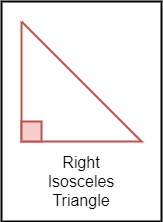Exercise: One angle is 90°, and two are equal, so what are the angles?

Solution:

There can't be two 90° angles, as then the total sum would be >180°, which is against the rules. Therefore, the two unknown angles will need to have a sum of 180° - 90° = 90°, meaning that each of them is 90° / 2 = 45°.

The study of triangles yields a whole new branch of mathematics, known as Trigonometry, for which there's already a complete series on this blog:

Thus, we will not get in-depth into such topics in the Geometry series...

A quadrilateral is a shape of four sides and four vertices.
It can also be called a Quadrangle (four angles) or a Tetragon (four polygon).
Its interior angles always add up to 360°.
Similar to triangles, quadrilaterals are also given special names.
Thus, we have the following four-sided shapes: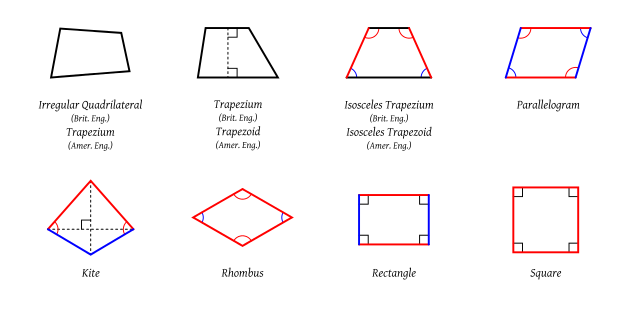[Image 3]

• Rectangle: All angles are equal and of 90°. Opposite sides are equal length.
• Square: All angles are equal (90°) and all sides have equal length.
• Rhombus: All sides have equal length. Opposite sides are parallel and opposite angles are equal.
• Parallelogram: Opposite sides parallel and equal length. Opposite angles equal. (Note: Squares, Rectangles and Rhombuses are all Parallelograms).
• Trapezoid: A pair of opposite sides is parallel.
• Isosceles Trapezoid: A trapezoid with left and right sides of equal length, which join to the base at equal angles.
• Trapezium: No parallel sides.
• Kite: Two pairs of sides, which are made of two equal-length sides that join up.
• Irregular: Only the square is a regular shape, the other quadrilaterals are irregular shapes.

Note: In The U.K. the trapezoid-trapezium definitions are inverted!

Exercise: Is a Square also an Isosceles Trapezoid?

Solution:

Yes, it is, because at least two opposite sides are parallel and the other two join the base at equal angles.

### Perimeter

The perimeter is the distance around a two-dimensional shape.
Here's how it can be calculated for various common shapes:

• Triangle: Sum of the sides: Perimeter = a + b + c
• Quadrilateral: Sum of the sides: Perimeter = a + b + c + d
• Square: If a is the length of one side: Perimeter = 4 x a
• Rectangle: If a and b are the lengths of the opposing sides: Perimeter = 2 x (a + b)
• Circle: If r is the circle's radius then the so called Circumference = 2πr

### Area

The area is basically the size of the surface of a shape.
Here's how it can be calculated for various common shapes:

• Triangle: Half of the base (b) times the height measured at right angles to the base (h): Area = ½ x b x h
• Square: If a is the length of one side: Area =
• Rectangle: If w and h are the width and height correspondingly: Area = w x h
• Parallelogram: If b and h are the base and vertcal height correspondingly: Area = b x h
• Circle: If r is the circle's radius: Area = π x r²

### Circles

A circle can be defined by a center point and a radius.
Thus, the shortest measure is the radius. The diameter is the longest measure and going striaght across the circle through its center. The diameter is exactly twice the radius.

For any circle it's circumference divided by the diameter yields the number π. Or we could also say that a circle with a diameter of 1 has a circumference of π.

The various lines that pass through or "touch" circles are given special names.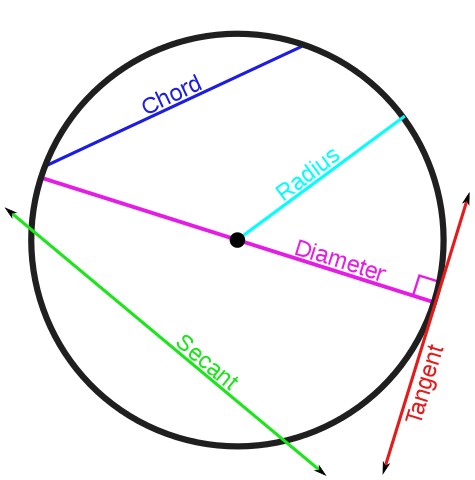[Image 4]

• Tangent: a line that "just touches" the circle at a specific point.
• Secant: a line that cuts the circle at two points.
• Chord: a line segment that goes from one point to another on the circle's circumference.
• Diameter: a chord that passes through the circle's center

A part of the circumference is called an Arc. Slicing the circle yields either Sectors or Segments. The Semicircle and Quadrant are special types of sectors.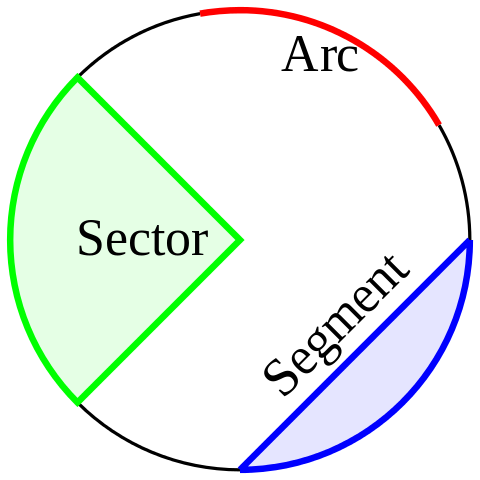[Image 5]

## RESOURCES:

### Images

Block diagrams and other visualizations were made using draw.io

## Final words | Next up

And this is actually it for today's post!

Next time we will continue with part 2.

Also, currently, other ideas for "All About" articles that I have in mind include:

• Polynomial Arithmetic
• Exponentials and Logarithms
• Rational Expressions

Basically more High-School Math Refreshers!

See ya!Keep on drifting!